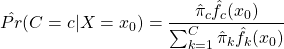## Motivation

For observed pairs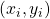,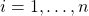, the relationship between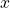and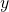can be defined generally as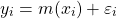where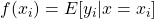and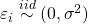. If we are unsure about the form of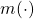, our objective may be to estimatewithout making too many assumptions about its shape. In other words, we aim to “let the data speak for itself”.

Simulated scatterplot of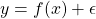. Here,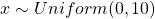and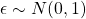. The true function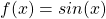is displayed in green.

Non-parametric approaches require only thatbe smooth and continuous. These assumptions are far less restrictive than alternative parametric approaches, thereby increasing the number of potential fits and providing additional flexibility. This makes non-parametric models particularly appealing when prior knowledge about‘s functional form is limited.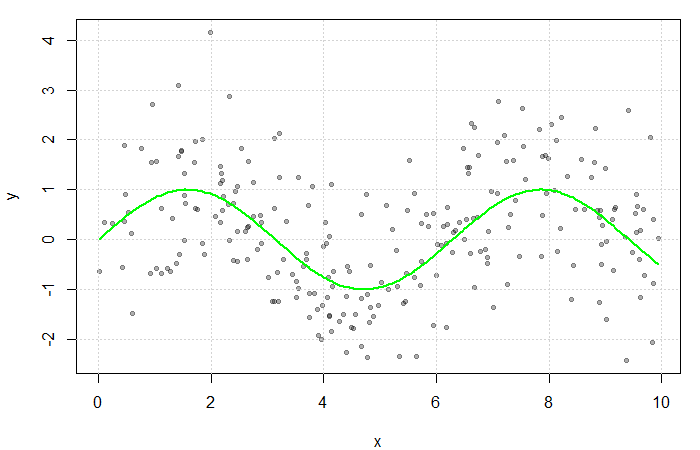## Estimating the Regression Function

If multiple values ofwere observed at each,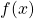could be estimated by averaging the value of the response at each. However, sinceis often continuous, it can take on a wide range of values making this quite rare. Instead, a neighbourhood ofis considered.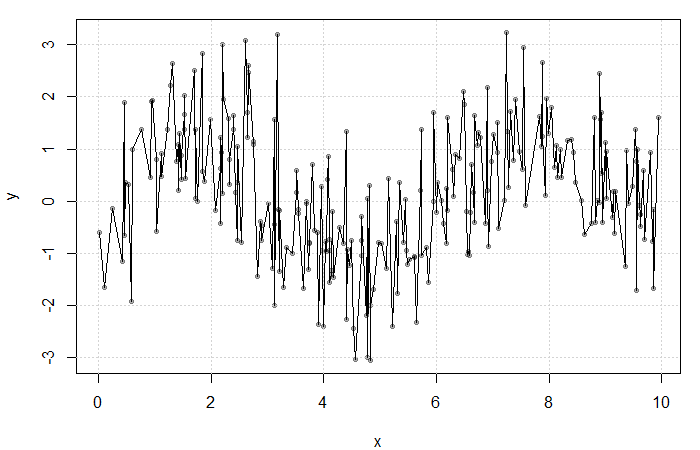Result of averaging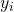at each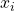. The fit is extremely rough due to gaps inand lowfrequency at each.

Define the neighbourhood aroundas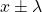for some bandwidth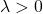. Then, a simple non-parametric estimate of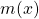can be constructed as average of the‘s corresponding to thewithin this neighbourhood. That is,

(1)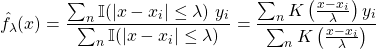where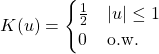is the uniform kernel. This estimator, referred to as the Nadaraya-Watson estimator, can be generalized to any kernel function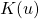(see my previous blog bost). It is, however, convention to use kernel functions of degree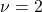(e.g. the Gaussian and Epanechnikov kernels).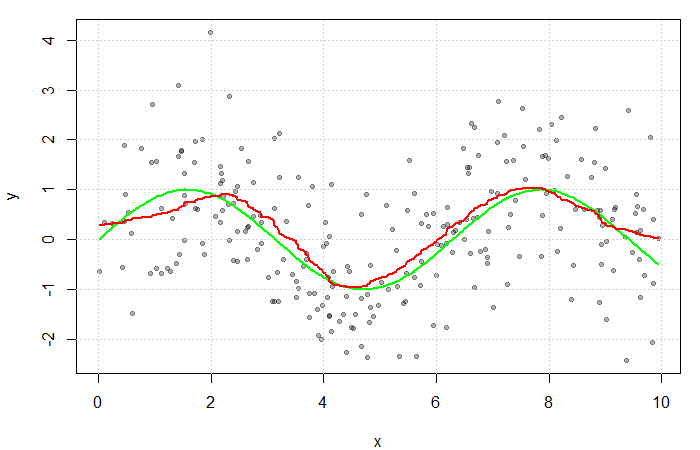The red line is the result of estimatingwith a Gaussian kernel and arbitrarily selected bandwidth of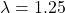. The green line represents the true function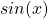.

## Motivation

It is important to have an understanding of some of the more traditional approaches to function estimation and classification before delving into the trendier topics of neural networks and decision trees. Many of these methods build on an understanding of each other and thus to truly be a MACHINE LEARNING MASTER, we’ve got to pay our dues. We will therefore start with the slightly less sexy topic of kernel density estimation.

Let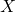be a random variable with a continuous distribution function (CDF)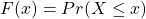and probability density function (PDF)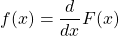Our goal is to estimatefrom a random sample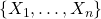. Estimation ofhas a number of applications including construction of the popular Naive Bayes classifier,